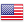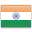Grade "A+" Accredited by NAAC with a CGPA of 3.46
Grade "A+" Accredited by NAAC with a CGPA of 3.46

# Artificial Intelligence

Course ID
BHCS 13
Level
Program
B.Sc. CS (Hons.)
Semester
Sixth
Credits
6.0
Paper Type
Core Course
Method
Lecture & Practical

## Unique Paper Code: Update Awaited

This course introduces the basic concepts and techniques of Artificial Intelligence (AI). The course aims to introduce intelligent agents and reasoning, heuristic search techniques, game playing, knowledge representation, reasoning with uncertain knowledge.

## Learning Outcomes:

At the end of the course, students should be able to:

• Identify problems that are amenable to solution by specific AI methods.
• Represent knowledge in Prolog and write code for drawing inferences.
• Identify appropriate AI technique for the problem at hand.
• Compare strengths and weaknesses of different artificial Intelligence techniques.
• Sensitive towards development of responsible Artificial Intelligence.

## Course Contents

Unit 1
Unit 2
Unit 3
Unit 4
Unit 5
Unit 6
Unit 7

Unit 1

Introduction: Introduction to artificial intelligence, background and applications, Turing test, rational agents, intelligent agents, structure, behaviour and environment of intelligent agents.

Unit 2

Knowledge Representation: Propositional logic, first order predicate logic, resolution principle, unification, semantic nets, conceptual dependencies, frames, scripts, production rules, conceptual graphs.

Unit 3

Reasoning with Uncertain Knowledge: Uncertainty, non-monotonic reasoning, truth maintenance systems, default reasoning and closed world assumption, Introduction to probabilistic reasoning, Bayesian probabilistic inference, introduction to fuzzy sets and fuzzy logic, reasoning using fuzzy logic.

Unit 4

Problem Solving and Searching Techniques: Problem characteristics, production systems, control strategies, breadth first search, depth first search, hill climbing and its variations, heuristics search techniques: best first search, A* algorithm, constraint satisfaction problem, means-end analysis.

Unit 5

Game Playing: introduction to game playing, min-max and alpha-beta pruning algorithms. Prolog Programming: Introduction to Programming in Logic (PROLOG), Lists, Operators, basic Input and Output.

Unit 6

Understanding Natural Languages: Overview of linguistics, Chomsky hierarchy of grammars, parsing techniques.

Unit 7

Ethics in AI, Fairness in AI, Legal perspective

### Practicals

#### Lab List 1

1. Write a prolog program to calculate the sum of two numbers.
2. Write a Prolog program to implement max(X, Y, M) so that M is the maximum of two numbers X and Y.
3. Write a program in PROLOG to implement factorial (N, F) where F represents the factorial of a number N.
4. Write a program in PROLOG to implement generate_fib(N,T) where T represents the Nth term of the fibonacci series.
5. Write a Prolog program to implement GCD of two numbers.
6. Write a Prolog program to implement power (Num,Pow, Ans) : where Num is raised to the power Pow to get Ans.
7. Prolog program to implement multi (N1, N2, R) : where N1 and N2 denotes the numbers to be multiplied and R represents the result.
8. Write a Prolog program to implement memb(X, L): to check whether X is a member of L or not.
9. Write a Prolog program to implement conc (L1, L2, L3) where L2 is the list to be appended with L1 to get the resulted list L3.
10. Write a Prolog program to implement reverse (L, R) where List L is original and List R is reversed list.

#### Lab List 2

1. Write a program in PROLOG to implement palindrome (L) which checks whether a list L is a palindrome or not.
2. Write a Prolog program to implement sumlist(L, S) so that S is the sum of a given list L.
3. Write a Prolog program to implement two predicates evenlength(List) and oddlength(List) so that they are true if their argument is a list of even or odd length respectively.
4. Write a Prolog program to implement nth_element (N, L, X) where N is the desired position, L is a list and X represents the Nth element of L.
5. Write a Prolog program to implement maxlist(L, M) so that M is the maximum number in the list.
6. Write a prolog program to implement insert_nth (I, N, L, R) that inserts an item I into Nth position of list L to generate a list R.
7. Write a Prolog program to implement delete_nth (N, L, R) that removes the element on Nth position from a list L to generate a list R.
8. Write a program in PROLOG to implement merge (L1, L2, L3) where L1 is first ordered list and L2 is second ordered list and L3 represents the merged list.

#### Text Books

Rich, E. & Knight,K. (2012). Artificial Intelligence. 3rd edition. Tata McGraw Hill.
Russell, S.J. & Norvig, P. (2015) Artificial Intelligence – A Modern Approach. 3rd edition. Pearson Education

Bratko, I. (2011). Prolog Programming for Artificial Intelligence. 4th edition. Pearson Education
Clocksin, W.F. & Mellish (2003), Programming in PROLOG. 5th edition. Springer
Kaushik, S. (2011). Artificial Intelligence. Cengage Learning India.
Patterson, D.W. (2015). Introduction to Artificial Intelligence and Expert Systems. 1st edition. Pearson Education.

#### Web Resources

https://cyber.harvard.edu/topics/ethics-and-governance-ai
https://royalsocietypublishing.org/doi/full/10.1098/rsta.2018.0085
https://arxiv.org/abs/1812.02953

#### Teaching Learning Process

Use of ICT tools in conjunction with traditional class room teaching methods
Interactive sessions
Class discussions

#### Assessment Methods

Written tests, assignments, quizzes, presentations as announced by the instructor in the class

#### Keywords

Artificial Intelligence, Problem Solving, Knowledge Representation, Reasoning, Uncertainty, Natural Language Processing.

Disclaimer: Details on this page are subject to change as per University of Delhi guidelines. For latest update in this regard please refer to the University of Delhi website here.Englishहिन्दी